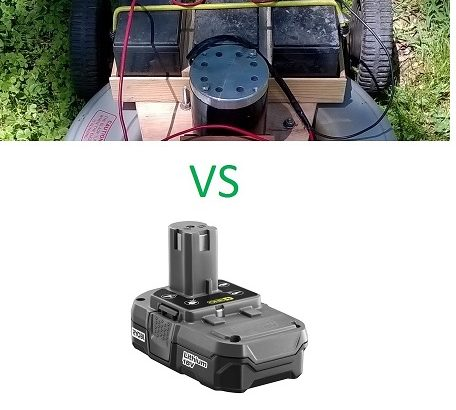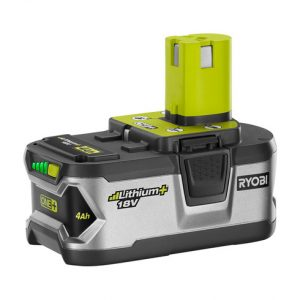Electric lawnmower tests lead acid vs lithium ion batteries initial conclusions, Apples vs Oranges, Battery technology evolving rapidly, Amps vs volts

Here are my initial findings and conclusions from testing the electric lawnmower I built first using 3 12v lead acid batteries and then 2 18v Lithium Ion batteries.

The batteries are from the same era, at least 7 years ago.

The Lithium Ion batteries however were used much.

Both sets of batteries, were connected in series to provide 36v required by the motor.

There was a noticeable difference in power, which after reflection came as no surprise.

The Lithium Ion batteries had a reduction in power due to much use but even if they were new, they had less Amp capacity than the lead acid batteries.

However, battery technology has evolved rapidly and Ryobi now has the 18v battery with at least twice the amp capacity rated at 4Ah. I will purchase these (or something even better) sometime in the future and retest.Here is a good explanation about the meaning of volts, amps and watts.

From Owlcation.

## “Amps

Electricity consists of the flow of electrons through a conductor such as an electric wire. You can’t see electrons but a helpful analogy is to think of electricity as the flow of water through a pipe.

We measure the rate of flow of electricity as an electric current (just as we think of the rate of flow of water in a river as the river current). The letter used to represent current in an equation is I.

Electric current is measured in Amperes, shortened to Amps or simply the letter A.

A current of 2 Amps can be written as 2A. The bigger the current the more electricity is flowing.

## Volts

So what makes the current flow in the first place? A device such as a battery provides what is known as a potential difference in an electric circuit.

If we go back to our water analogy, the battery is like a water pump that propels water through a pipe.

It creates pressure in the pipe causing the water to flow. So how do we measure this pressure? We call electrical pressure Voltage and measure it in Volts, shortened to V. The letter V is also used represent Voltage in an equation.

A voltage of 3 volts can be written as 3V.

The bigger the voltage the higher the pressure and the more current flows. But in order for the current to flow, the electrical conductor or wire must loop back to the battery.”

## “Watts

So how do Current and Voltage relate to one another? Well, the bigger the current the brighter the light and similarly the bigger the voltage the brighter the light.

Both the voltage and the current in the bulb determine how much energy is released in a certain time.

The Watt is a measure of power or how much energy is released per second. It can be shortened to W.

1 Watt can be written 1W.

We can calculate the power released in a bulb by multiplying the voltage in Volts across the bulb by the current in Amps flowing through the bulb (W = V x I).

For example a current of 2 Amps flowing through a bulb with 12 Volts across it generates 24 watts of power.”